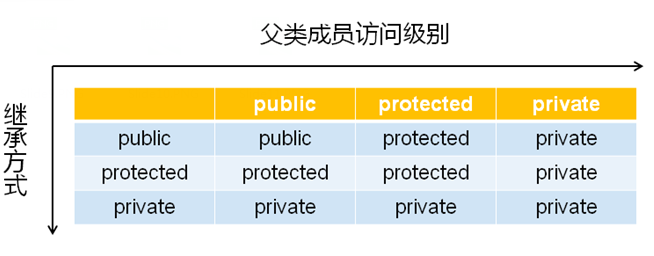# 上章链接: 22.C++- 继承与组合,protected访问级别

```class Line : public Object             //继承方式是public
{
};```

public继承

-指父类的成员(变量和函数)访问级别,在子类中保持不变

private继承

-指父类的成员,在子类中变为private私有成员.

-也就是说子类无法访问父类的所有成员

protected继承

-指父类的public成员 ,在子类中变为protected保护成员,其它成员级别保持不变• 当我们创建子类对象时,编译器会默认调用父类无参构造函数
• 若有子类对象,也会默认调用子类对象的无参构造函数。

```class  StrA
{
public:
StrA()
{
cout<<"StrA()"<<endl;
}
StrA(string s)
{
cout<<"StrA(string s):"<<s<<endl;
}
};

class  StrB : public StrA
{
public:
StrB(string s)
{
cout<<"StrB(int i):"<<s<<endl;
}
};

int main()
{
StrB b("123");
return 0;
}```

编译运行:

```StrA()                    //父类无参构造函数
StrB(int i):123```

```StrB(string s): StrA(s)
{
cout<<"StrB(int i):"<<s<<endl;
}```

```StrA(string s):123
StrB(int i):123```

• 子类可以定义父类中的同名成员和同名函数
• 子类中的成员变量和函数将会隐藏父类的同名成员变量和函数
• 父类中的同名成员变量和函数依然存在子类中
• 通过作用域分辨符（::）才可以访问父类中的同名成员变量和函数

```class Parent{

public:
int mval;
Parent()
{
mval=1000;
}
{
mval+=i;
}
};

class Child : public Parent
{
public:
int mval;
Child()
{
mval=100;
}

{
mval+=i+j;
}
};```

```       Child c;

cout<<"Child.mval="<<c.mval<<endl;

cout<<"Parent.mval="<<c.Parent::mval<<endl;```

```Child.mval=105
Parent.mval=1010```

• 子类对象可以直接赋值给父类对象使用,比如: Parent p; Child c;   p=c;
• 子类对象可以初始化父类对象,比如: Parent p1(c);
• 父类引用可以直接引用子类对象,比如: Parent& p2 =c;    //p2是c对象的别名
• 父类指针可以直接指向子类对象,比如: Parent* p3=&c;

```Child c;
Parent* p3=&c;
Child *c2 = (Child*)p3;         ```

```Child c;
Parent* p3=&c;
Child *c2 = (static_cast*)<Child*>(p3);```

```class Parent
{
int i;
public:
void example()
{
cout<<"class Parent"<<endl;
}

};

class Child : public Parent
{
int j;
public:
void example()
{
cout<<"class Child"<<endl;
}
};

void print(Parent* p)
{
p->example();
}
int main()
{
Parent t;
Child c;

print(&t);
print(&c);

cout<<"SIZEOF Parent:"<<sizeof(t)<<endl;
cout<<"SIZEOF Child:"<<sizeof(c)<<endl; }```

```class Parent
class Parent
SIZEOF Parent:4
SIZEOF Child:8```

```virtual void print()        //将父类的成员函数定为虚函数
{
cout<<"class Parent"<<endl;
}        ```

```class Parent
class Child
SIZEOF Parent:8
SIZEOF Child:12```

-在使用基类指针指向派生类对象时用到

-通过基类析构函数可以删除派生类对象

```#include <iostream>

using namespace std;

class Base
{
public:
Base()
{
cout << "Base()" << endl;
}

virtual ~Base()
{
cout << "~Base()" << endl;
}
};

class Derived : public Base
{
public:
Derived()
{
cout << "Derived()" << endl;
}

~Derived()
{
cout << "~Derived()" << endl;
}
};

int main()
{
Base* p = new Derived();
// ...
delete p;

return 0;
}```

```Base()
Derived()
~Derived()
~Base()```